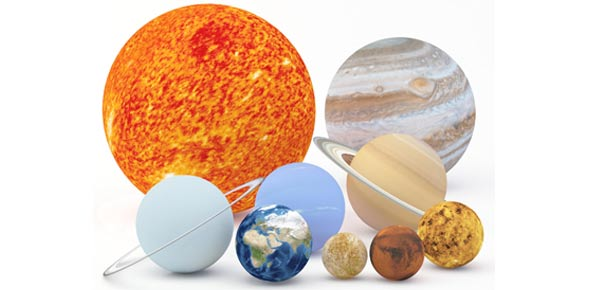# Astronomy Terms Quiz

19 Questions | Total Attempts: 132SettingsA quiz on astronomical terms. Reasonably hard at times and 20 questions long. Also you only get a half minute per question.

Related Topics
• 1.
What is the process by which small particles coalesce to form planets or satellites?
• A.

Oblate

• B.

Conjunction

• C.

Combination

• D.

Accretion

• E.

Declination

• 2.
What is the point 90 degrees from the horizon directly above an observer referred to?
• A.

• B.

Parsec

• C.

Syzygy

• D.

Solstice

• E.

Zenith

• 3.
The study of how the universe was formed is what?
• 4.
The study of the properties, processes, and evolution of the universe is what?
• 5.
What is a pattern of stars that is not a constellation known as?
• A.

Group

• B.

Nebula

• C.

Asterism

• D.

Sidereal

• E.

Umbra

• 6.
Most planets appear to spin eastward, like the earth.  However some don't and that apparent westward rotation is known as -------- motion
• A.

Synchronous

• B.

Parallax

• C.

Prominent

• D.

• E.

Backward

• 7.
The angle of an object eastward from  the vernal equinox along the celestial equator and is the equivalent of celestial longitude is what?
• A.

Right ascension

• B.

Left ascension

• C.

Parallax

• D.

Eclptic

• E.

Declination

• 8.
What is the full name of the H-R Diagram
• A.

• B.

Hertzsprung-Russell Diagram

• C.

Halley-Russell Diagram

• D.

Hawking-Ryans Diagram

• E.

Herschel-Roberts Diagram

• 9.
What term gives the distance from the center of a collapsing star where not even light can escape from the gravitational pull? (two words)
• 10.
True or False? Spring tides occur at the new moon and full moon most frequently.
• 11.
What is the minimum required mass of a black hole? (in solar masses)
• 12.
What is the point in a black hole where space and time are infinitely distorted and the density is infinite (for all mathmatical purposes) known as?
• 13.
What is the time it takes a the moon to complete one cycle of its phases as seen from earth known as?  (not a number of days or "lunar")
• 14.
Which is the word that has the least relation to the others?
• A.

Solar

• B.

Umbra

• C.

Albedo

• D.

Lunar

• E.

Eclipse

• 15.
What is the distance from a planet where the planet's gravity will literally tear a certain object apart is known as what?
• 16.
IsWhich of Kepler's laws says that an imaginary line joining a planet to the sun sweeps out equal areas of an ellipse over equal intervals of time?
• A.

1st

• B.

2nd

• C.

3rd

• D.

4th

• E.

5th

• 17.
Which of Kepler's laws says that each planet moves about the Sun in an orbit that is an ellipse with the Sun at one of the foci of said ellipse?
• A.

1st

• B.

2nd

• C.

3rd

• D.

4th

• E.

5th

• 18.
Which of Kepler's laws says that the square of the period of revolution of a planet is in direct proportion to the cube of the semimajor axis of its orbit?
• A.

1st

• B.

2nd

• C.

3rd

• D.

4th

• E.

5th

• 19.
Name one of the 5 major layers of the sun